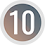# Algebraic Expressions

## Algebraic Expressions

A mathematical statement which is obtained by using mathematical fundamental operation between constants and variables with different powers is called an algebraic expression.

********************

10 Math Problems officially announces the release of Quick Math Solver, an Android App on the Google Play Store for students around the world.

********************

For example:
-     The sum of 2 and x is 2 + x, where the constant 2 and variable x is connected by ‘+’ sign.
-     So, 2 + x is an algebraic expression.
-     Similarly, other algebraic expressions are 2xy, 3x + 4y, x – y2 + 4 , etc.

### Algebraic Terms

The parts of an algebraic expression separated by ‘+’ or ‘–’ signs are called algebraic terms.

For example:
-     3x + 2y is an algebraic expression, where 3x and 2y are two parts connected by ‘+’ sign. So, 3x and 2y are called the terms of the expression 2x + 3y.

### Types of algebraic expression

Algebraic expressions are of different types. They are: Monomial, Binomial, Trinomial and multinomial expressions.

-     Monomial Expression: An algebraic expression which contains only one term is called a monomial expression. For example: 4x, 3x2y, -7xyz2, etc.

-     Binomial Expression: An algebraic expression which contains two terms connected by ‘+’ or ‘–’ is called a binomial expression. For example: a + b, 2a2 – 3abx2, 3x – 4ay2, etc.

-     Trinomial Expression: An algebraic expression which contains three terms connected by ‘+’ or ‘–’ is called a trinomial expression. For example: x + 2y – z, a2 + 2ab + b2, etc.

-     Multinomial Expression: An algebraic expression which contains four or more than four terms connected by ‘+’ or ‘–’ is called a multinomial expression. For example: 2x – 2y + z, x3 – a2 + 2ab – b2 + 7, etc.

### Coefficient, base and power

-     Coefficient: A numerical or constant quantity placed before the variable and multiplying it is called the coefficient. For example: In an expression 3x, 3 is called the coefficient of x.

-     Base: An alphabet letter used in algebraic expression is called the base. For example: In an expressioin 3x2, x is the base.

-     Power: The repeatation of the same variable for the required number of times in an algebraic expression is called power. For example: In an expressioin 3x2, 2 is the power of x.### Like and Unlike Terms

-     Like Terms: The terms are like if the terms have the same base with same power of the variable. For example: 2a, -3a, 4a are like terms. x3, 2x3, -5x3, -3x3 are like terms. similarly 7xy, -3xy, 2xy are like terms.

-     Unlike Terms: The terms are unlike if the terms have the different variables or different powers of the base. For example: x, 2x2, 3x2y, -y are unlike terms. x3, x4, x-3, -x are unlike terms. Similarly xy, 2xz, 3x2y are unlike terms.

### Values of Algebraic Expressions

When we substitute the value of a variable in an algebraic expression with a number, the value of the expression is obtained after use of mathematical fundamental operations, it is called the value of the expression.

For example: if x = 2 and y = 3, find the value of 2x – 3x2y.
Here, the given expression = 2x – 3x2y
= 2 × 2 – 3 × 22 × 3
= 4 – 3 × 4 × 3
= 4 – 36
= -32

### Workout Examples

Example 1: Write the type of each expression.

a.     4x
b.    2a + 3b
c.     3x2 – 4xy + 2y2
d.    9x3 – 10x2 + 4x – 21
e.     3x3 – 2x2 + 5x + 7xy - 8

Solution: Here,
a.     4x -------> Monomial
b.    2a + 3b ---------> Binomial
c.     3x2 – 4xy + 2y2 ---------> Trinomial
d.    9x3 – 10x2 + 4x – 21 ----------> Multinomial
e.     3x3 – 2x2 + 5x + 7xy – 8 ----------> Multinomial

Example 2: Rewrite the following statements in algebraic expressions.

a.     The sum of twice x and 5.
b.    Three times the difference of x and y is less than 3a.
c.     5x is added to the product of b and 7.
d.    One fourth of x is added to 25
e.     7x is divided by 14 and added to product of c and 4.

Solution: Here,
a.     2x + 5
b.    3(x – y) < 3a
c.     5x + 7b
d.    x/4 +25
e.     7x/14 +4c

Example 3: Write the terms having:

a.     Base = x, power = 2 and coefficient = 1
b.    Base = x, power = 3 and coefficient = -3
c.     Base = y, power = 5 and coefficient = 4b
d.    Base = z, power = 2 and coefficient = -a

Solution: Here,
a.     x2
b.    -3x3
c.     4by5
d.    –az2

Example 4: Identify the like and unlike terms of the following expressions:
a.     3x, -2x, 7x
b.    3a2, 3a3, 4a
c.     2(x+y), 3(x+y)3, 9(x+y)2

Solution: Here,
a.     Like terms
b.    Unlike terms
c.     Unlike terms

Example 5: If x = 4 and y = 3, find the value of 2xy + 3y2

Solution: Here,
x = 4
y = 3
2xy + 3y2 = 2 × 4 ×3 + 3 × 32
= 24 + 3 × 9
= 24 + 27
= 51

You can comment your questions or problems regarding the algebraic expressions here.

1.1.